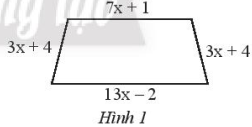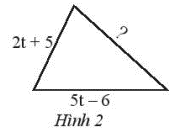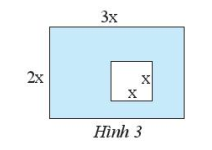## Solving SBT Lesson 3: One-variable polynomial addition and subtraction (C7 SBT Math 7 Horizons) – Math Book

Solving SBT Lesson 3: One-variable polynomial addition and subtraction (C7 SBT Math 7 Horizon)
———

### Solution 1 page 30 SBT Math 7 Creative horizon episode 2 – CTST

Given two polynomials $$P\left( x \right) = – 4{x^4} – 3{x^2} + 7$$ and $$Q\left( x \right) = 2{x^4 } – 5{x^2} + 8x – 1$$. Calculate $$P\left( x \right) + Q\left( x \right)$$ and $$P\left( x \right) – Q\left( x \right)$$.

Detailed instructions for solving Lesson 1

Solution method

Step 1: Add and subtract mononomials of the same variable to reduce the given polynomial.

Step 2: Sort the monomials by the descending power of the variable.

Step 3: Perform the calculation horizontally or vertically.

Detailed explanation

$$P\left( x \right) + Q\left( x \right) = – 4{x^4} – 3{x^2} + 7 + 2{x^4} – 5{x^2} + 8x – 1 = – 2{x^4} – 8{x^2} + 8x + 6$$

$$P\left( x \right) – Q\left( x \right) = – 4{x^4} – 3{x^2} + 7 – \left( {2{x^4} – 5{ x^2} + 8x – 1} \right) = – 6{x^4} + 2{x^2} – 8x + 8$$.

–>

— *****

### Solve problem 2 page 30 SBT Math 7 Creative horizon episode 2 – CTST

Let the polynomial $$A\left( t \right) = 2{t^4} – 8{t^3} + 9t + 3$$. Find the polynomial $$B\left( t \right)$$ such that $$B\left( t \right) – A\left( t \right) = – 4{t^3} + 3{t^2 } + 8t$$.

Detailed instructions for solving Lesson 2

Solution method

Step 1: Add and subtract mononomials of the same variable to reduce the given polynomial.

Step 2: Sort the monomials by the descending power of the variable.

Step 3: Perform the calculation horizontally or vertically.

Detailed explanation

$$\begin{array}{l}B\left( t \right) – A\left( t \right) = – 4{t^3} + 3{t^2} + 8t \Rightarrow B\left( t \right) = – 4{t^3} + 3{t^2} + 8t + A\left( t \right)\\ = – 4{t^3} + 3{t^2} + 8t + 2{t^4} – 8{t^3} + 9t + 3 = 2{t^4} – 12{t^3} + 3{t^2} + 17t + 3\end{array}$$

So $$B\left( t \right) = 2{t^4} – 12{t^3} + 3{t^2} + 17t + 3$$.

–>

— *****

### Solution 3 page 30 SBT Math 7 Creative horizon episode 2 – CTST

Let the polynomial $$M\left( x \right) = 4{x^3} – 7{x^2} + 2x – 9$$. Find the polynomial $$N\left( x \right)$$ such that $$M\left( x \right) + N\left( x \right) = 2{x^3} – 6x$$.

Detailed instructions for solving Lesson 3

Solution method

Step 1: Add and subtract mononomials of the same variable to reduce the given polynomial.

Step 2: Sort the monomials by the descending power of the variable.

Step 3: Perform the calculation horizontally or vertically.

Detailed explanation

We have $$M\left( x \right) + N\left( x \right) = 2{x^3} – 6x \Rightarrow N\left( x \right) = 2{x^3} – 6x – M\left( x \right) = 2{x^3} – 6x – \left( {4{x^3} – 7{x^2} + 2x – 9} \right)$$$$– 2{ x^3} + 7{x^2} – 8x + 9$$

So $$N\left( x \right) = – 2{x^3} + 7{x^2} – 8x + 9$$

–>

— *****

### Solution 4 page 30 SBT Math 7 Creative horizon episode 2 – CTST

Given three polynomials $$P\left( x \right) = 3{x^4} – 2{x^2} + 8x – 10$$; $$Q\left( x \right) = 4{x^3} – 6{x^2} + 7x – 1$$ and $$R\left( x \right) = – 3{x^4} + 5{x^2} – 8x – 5$$. Calculate $$P\left( x \right) + Q\left( x \right) + R\left( x \right)$$ and $$P\left( x \right) – Q\left( x \right ) – R\left( x \right)$$.

Detailed instructions for solving lesson 4

Solution method

Step 1: Add and subtract mononomials of the same variable to reduce the given polynomial.

Step 2: Sort the monomials by the descending power of the variable.

Step 3: Perform the calculation horizontally or vertically.

Detailed explanation

$$\begin{array}{*{20}{c}}{}&{3{x^4}}&{}&{}& – &{2{x^2}}& + &{8x} & – &{10}\\ + &{}&{}&{}&{}&{}&{}&{}&{}&{}\\{}&{}&{}&{4{ x^3}}& – &{6{x^2}}& + &{7x}& – &1\\ + &{}&{}&{}&{}&{}&{}&{}& {}&{}\\{}&{ – 3{x^4}}&{}&{}& + &{5{x^2}}& – &{8x}& – &5\\\hline{ }&{}&{}&{4{x^3}}& – &{3{x^2}}& + &{7x}& – &{16}\end{array}$$

So $$P\left( x \right) + Q\left( x \right) + R\left( x \right) = 4{x^3} – 3{x^2} + 7x – 16$$

$$\begin{array}{*{20}{c}}{}&{3{x^4}}&{}&{}& – &{2{x^2}}& + &{8x} & – &{10}\\ – &{}&{}&{}&{}&{}&{}&{}&{}&{}\\{}&{}&{}&{4{ x^3}}& – &{6{x^2}}& + &{7x}& – &1\\ – &{}&{}&{}&{}&{}&{}&{}& {}&{}\\{}&{ – 3{x^4}}&{}&{}& + &{5{x^2}}& – &{8x}& – &5\\\hline{ }&{6{x^4}}& – &{4{x^3}}& – &{{x^2}}& + &{9x}& – &4\end{array}$$

So $$P\left( x \right) – Q\left( x \right) – R\left( x \right) = 6{x^4} – 4{x^3} – {x^2} + 9x – 4$$.

–>

— *****

### Solve problems 5 pages 30 SBT Math 7 Creative horizon episode 2 – CTST

Let the polynomial $$P\left( x \right) = – 3{x^2} + 7x – 5$$. Write $$P\left( x \right)$$ as the sum of two quadratic polynomials.

Detailed instructions for solving Lesson 5

Solution method

The degree of the polynomial $$P\left( x \right)$$ is 2, so to write $$P\left( x \right)$$ as the sum of two quaternions, the two quaternions must have coefficients of opposite sign

Detailed explanation

$$P\left( x \right) = \left( {2{x^4} + 4{x^2} + 2x – 1} \right) + \left( { – 2{x^4} – 7 {x^2} + 5x – 4} \right) = – 3{x^2} + 7x – 5$$

–>

— *****

### Solve lesson 6 pages 30 SBT Math 7 Creative horizon episode 2 – CTST

Write an expression for the perimeter of the isosceles trapezoid in Figure 1.Detailed instructions for solving Lesson 6

Solution method

Use the formulas learned about the perimeter of a trapezoid to write an expression.

Detailed explanation

The perimeter of a trapezoid is equal to the sum of the four sides. Hence we have the expression

$$7x + 1 + 3x + 4 + 13x – 2 + 3x + 4 = 26x + 7$$.

–>

— *****

### Solution 7 page 30 SBT Math 7 Creative horizon episode 2 – CTST

Given a triangle (see Figure 2) with perimeter $$12t – 6$$, Find the unknown side of that triangleDetailed instructions for solving Lesson 7

Solution method

The perimeter of a triangle is equal to the sum of its three sides. So, if we want to find the unknown edge, we subtract the known edges from the perimeter.

Detailed explanation

The expression for the unknown edge is:

$$12t – 6 – \left( {2t + 5} \right) – \left( {5t – 6} \right) = 5t – 5$$.

–>

— *****

### Solve problems 8 pages 30 SBT Math 7 Creative horizon episode 2 – CTST

Write an expression representing the area of ​​the shaded part in Figure 3.Detailed instructions for solving Lesson 8

Solution method

Use the learned area formulas to write the expression.

Area of ​​a rectangle is the product of length and width

The area of ​​the square is equal to the side squared.

Detailed explanation

The area of ​​the rectangle is $$2x.3x = 6{x^2}$$

Area of ​​square is $${x^2}$$

The area of ​​the shaded part is $$6{x^2} – {x^2} = 5{x^2}$$.

–>

— *****

### Solve problem 9 page 31 SBT Math 7 Creative horizon episode 2 – CTST

The number of passenger cars sold in a country from 1983 to 1996 is described by the formula $$C = – 0.016{t^4} + 0.49{t^3} – 4.8{t^ 2} + 14t + 70$$ (in thousands of units), while the number of trucks is in $$T = – 0.01{t^4} + 0.31{t^3} – 3 {t^2} + 11t + 23$$, where t is the number of years since 1983. Write an expression that represents the number of vehicles (both passenger cars and trucks) sold during the above period. Calculate the number of cars sold in 1990 (corresponding to $$t = 7$$).

Detailed instructions for solving Lesson 9

Solution method

Step 1: Arrange the mononomials of the two polynomials in order of decreasing (or ascending) power of the variable.

Step 2: Perform the calculation horizontally or vertically.

Detailed explanation

The expression for the number of vehicles (both passenger cars and vans) sold between 1983 and 1996 is

$$\begin{array}{l}C + T = – 0.016{t^4} + 0.49{t^3} – 4.8{t^2} + 14t + 70 + \left( { – 0 ,01{t^4} + 0.31{t^3} – 3{t^2} + 11t + 23} \right)\\ = \left( { – 0.016 – 0.01} \right){t ^4} + \left( {0.49 + 0.31} \right){t^3} + \left( { – 4.8 – 3} \right){t^2} + \left( {14 + 11} \right)t + 70 + 23\\ = – 0.026{t^4} + 0.8{t^3} – 7.8{t^2} + 25t + 93\end{array}$$

So $$C + T = – 0.026{t^4} + 0.8{t^3} – 7.8{t^2} + 25t + 93$$

Number of cars sold in 1990 (corresponding to $$t = 7$$) is

$$C + T = – 0,{026.7^4} + 0,{8.7^3} – 7,{8.7^2} + 25.7 + 93 = 97,774$$
So the number of cars sold in 1990 was 97774 units.

–>

— *****

### Solution 10 page 31 SBT Math 7 Creative horizon episode 2 – CTST

The population of the United States from 1980 to 1996 was calculated using the following formula:

$$P = – 0.8{t^4} + 27{t^3} – 262{t^2} + 3010t + 227000$$

And the number of people aged 85 and over is calculated by the formula:

$$S = 0.02{t^4} – 0.7{t^3} + 6.4{t^2} + 213t + 7740$$

Where P, S are in thousands of people, t is the number of years since 1980. Write an expression for the number of Americans under 85 years old and calculate that number in 1995 (corresponding to $$t = 15$$) .

Detailed instructions for solving Lesson 10

Solution method

Perform one-variable polynomial subtraction

Step 1: Arrange the mononomials of the two polynomials in order of decreasing (or ascending) power of the variable.

Step 2: Perform the calculation horizontally or vertically.

Detailed explanation

The expression for the number of Americans under the age of 85 is

$$\begin{array}{l}P – S = – 0.8{t^4} + 27{t^3} – 262{t^2} + 3010t + 227000 – \left( {0.02{ t^4} – 0.7{t^3} + 6.4{t^2} + 213t + 7740} \right)\\ = – 0.82{t^4} + 27.7{t^3 } – 268.4{t^2} + 2797t + 219260\end{array}$$

When $$t = 15$$, the number of Americans under the age of 85 is

$$P – S = – 0,{82.15^4} + 27,{7.15^3} – 268,{4.15^2} + 2797.15 + 219260 = 252800$$ people.

–>

— *****